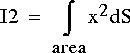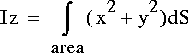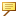Fundamentals > Fundamentals > Creo Parametric User Interface > The Analysis Tab > Analyzing Model Properties > List of Computed Mass Properties for a Cross Section
List of Computed Mass Properties for a Cross Section
The computed mass properties for a cross section include the following:
Surface Area—Area of the cross section.
Center of gravity—Location of the center of gravity with respect to a specified coordinate system. The center of gravity and coordinate system are displayed graphically on the model.
Inertia tensor at the specified coordinate system origin and axes.
Inertia tensor at the center of gravity and oriented along the specified coordinate system axes.
Principal moments of inertia—Moments of inertia at the center of gravity with respect to the principal axes.The principal coordinate axes are labeled 1 and 2 instead of x and y. In the preceding equations, x is the coordinate along principal axis 1 and y is the coordinate along principal axis 2.
Polar moment of inertia—Cross-sectional polar moment of inertia at the center of gravity.Rotation matrix and rotation angle—Rotation around the z-axis from the coordinate axes to the principal axes, in matrix and angular form.
Radii of gyration—The radii of gyration at the center of gravity with respect to the principal axes.
Section moduli and the corresponding points—Calculated for each principal axis (for example, 1-axis, 2-axis) by dividing the area moment of inertia with respect to the chosen axis by the distance to the point or points of the cross section that are farthest from the chosen axis. These points have the lowest (most negative) and highest (most positive) coordinate values along the orthogonal principal axis in the cross section plane.Cross section mass properties are not available for offset cross sections.